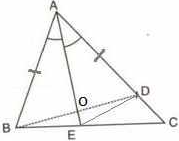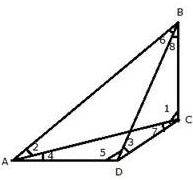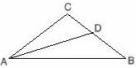# ICSE Solutions for Selina Concise Chapter 11 Inequalities Class 9 Maths

### Exercise 11

1. From the following figure, prove that: AB > CD.

In ΔABC,
AB = AC [Given]
∴ ∠ACB =  ∠B [angles opposite to equal sides are equal]
∠B = 70°  [Given]
⇒ ∠ACB = 70° ...(i)
Now,
∠ACB + ∠ACD = 180° [BCD is a straight line]
⇒ 70° + ∠ACD = 180°
⇒ ∠ACD = 110° ...(ii)
In ΔACD,
∠CAD + ∠ACD + ∠D = 180°
⇒ ∠CAD + 110° + ∠D = 180° [From (ii)]
⇒ ∠CAD + ∠D = 70°
But ∠D = 40°  [Given]
⇒ ∠CAD + 40° = 70°
In ΔACD,
∠ACD = 110° [From (ii)]
∠D = 40° [Given]
⇒ AC > CD [Greater angle has greater side opposite to it]
Also,
AB = AC [Given]
Therefore, AB > CD.

2. In a triangle PQR; QR = PR and ∠P = 36o. Which is the largest side of the triangle?

⇒∠Q = 36°
In ΔPQR,
∠P + ∠Q +∠R = 180°
⇒ 36° + 36° + ∠R = 180°
⇒ ∠R + 72° = 180°
⇒ ∠R = 108°
Now,
∠R = 108°
∠P = 36°
∠Q = 36°
Since ∠R is the greatest, therefore, PQ is the largest side.

3. If two sides of a triangle are 8 cm and 13 cm, then the length of the third side is between a cm and b cm. Find the values of a and b such that a is less than b.

The sum of any two sides of the triangle is always greater than third side of the triangle.

Third side < 13+8 =21 cm.

The difference between any two sides of the triangle is always less than the third side of the triangle.

Third side > 13-8 =5 cm.

Therefore, the length of the third side is between 5 cm and 9 cm, respectively.

The value of a =5 cm and b= 21cm.

4. In each of the following figures, write BC, AC and CD in ascending order of their lengths.In ΔABC,
AB = AC
⇒ ∠ABC = ∠ACB (angles opposite to equal sides are equal)
⇒ ∠ABC = ∠ACB = 67°
⇒ ∠BAC = 180° - ∠ABC - ∠ACB (angle sum property)
⇒ ∠BAC = 180° -67° - 67° = 46°
Since ∠BAC < ∠ABC, we have
BC < AC ...(1)
Now, ∠ACD = 180° - ∠ACB (linear pair)
⇒ ∠ACD = 180° - 67° = 113°
Thus, in ΔACD,
⇒ ∠CAD = 180° - 113° - 33° = 34°
AC < CD  ...(2)
From (1) and (2), we have
BC < AC< CD

In ΔABC,
∠BAC < ∠ABC
⇒BC < AC  ...(1)
Now, ∠ACB = 180° - ∠ABC - ∠BAC
⇒ ∠ACB = 180° - 73° - 47°
⇒ ∠ACB = 60°
Now, ∠ACD = 180° - ∠ACB
⇒ ∠ACD = 180° - 60° = 120°
Now, in ΔACD,
⇒ ∠ADC = 180° - 120° - 31°
AC < CD  ...(2)
From (1) and (2), we have
BC < AC < CD

5. Arrange the sides of ∆BOC in descending order of their lengths. BO and CO are bisectors of angles ABC and ACB respectively.

∠ABC = 180° - ∠ABE = 180° - 106° = 74°
Thus, in ΔABC,
∠ACB = 180° - ∠BAC - ∠ABC
⇒ ∠ACB = 180° - 43° - 74° = 63°
Now, ∠ABC = ∠OBC + ∠ABO
⇒ ∠ABC = 2∠OBC  (OB is bisector of ∠ABC)
⇒ 74° = 2∠OBC
⇒ ∠OBC = 37°
Similarly,
∠ACB = ∠OCB + ∠ACO
⇒ ∠ACB = 2∠OCB  (OC is bisector of ∠ACB)
⇒ 63° = 2∠OCB
⇒ ∠OCB = 31.5°
Now in ΔBOC,
∠BOC = 180° - ∠OBC - ∠OCB
⇒ ∠BOC = 180° - 37° - 31.5°
⇒ ∠BOC = 111.5°
Since, ∠BOC > ∠OBC > ∠OCB, we have
BC > OC > OB

6. D is a point in side BC of triangle ABC. If AD > AC, show that AB > AC.

Now, ∠ADC > ∠B + ∠BAC  (Exterior Angle Property)
From (1) and (2), we have
⇒ ∠C > ∠B
⇒ AB > AC

7. In the following figure, ∠BAC = 60o and ∠ABC = 65o.

Prove that:

(i) CF > AF

(ii) DC > DF

In ΔBEC,
∠B + ∠BEC + ∠BCE = 180°
∠B = 65°  [Given]
∠BEC = 90°  [CE is perpendicular to AB]
⇒ 65° + 90° + ∠BCE  = 180°
⇒ ∠BCE = 180° - 155°
⇒ ∠BCE = 25° = ∠DCF ...(i)
In ΔCDF,
∠DCF + ∠FDC + ∠CFD = 180°
∠DCF = 25°  [From (i)]
∠FDC = 90°  [AD is perpendicular to BC]
⇒ 25° + 90° + ∠CFD  = 180°
⇒ ∠CFD = 180° - 115°
⇒ ∠CFD = 65° ...(ii)
Now, ∠AFC + ∠CFD = 180° [AFD is a straight line]
⇒ ∠AFC + 65° = 180°
⇒ ∠AFC = 115° ...(iii)
In ΔACE,
∠ACE + ∠CEA + ∠BAC = 180°
∠BAC = 60°  [Given]
∠CEA = 90°  [CE is perpendicular to AB]
⇒ ∠ACE + 90° + 60° = 180°
⇒ ∠ACE = 180° - 150°
⇒ ∠ACE = 30° ...(iv)
In ΔAFC,
∠AFC + ∠ACF + ∠FAC = 180°
∠AFC = 115° [From (iii)]
∠ACF  = 30°  [From (iv)]
⇒ 115° + 30° + ∠FAC = 180°
⇒ ∠FAC = 180° - 145°
⇒ ∠FAC = 35° ...(v)
In ΔAFC,
∠FAC = 35° [From (v)]
∠ACF  =  30°  [From (iv)]
∴∠FAC > ∠ACF
⇒CF > AF
In ΔCDF,
∠DCF = 25°  [From (i)]
∠CFD = 65°  [From (ii)]
∴∠CFD > ∠DCF
⇒ DC > DF

8. In the following figure; AC = CD; ∠BAD = 110o and ∠ACB = 74o.

Prove that: BC > CD

∠ACB + ∠ACD = 180°  [BCD is a straight line]
⇒ 74° + ∠ACD = 180°
⇒ ∠ACD = 106° ...(ii)
In ΔACD,
Given that AC = CD
Now,
∠BAC + 37° = 110°
∠BAC = 73° ...(iv)
In ΔABC,
∠B + ∠BAC + ∠ACB = 180°
⇒ ∠B + 73° + 74° = 180°  [From (i) and (iv)]
⇒ ∠B + 147° = 180°
⇒ ∠B = 33° ...(v)
∴∠BAC > ∠B  [From (iv) and (v)]
⇒BC > AC
But,
AC = CD  [Given]
⇒ BC > CD

9. From the following figure; prove that:

(i) AB > BD

(ii) AC > CD

(iii) AB + AC > BC

Therefore, ∠B and ∠BAD are both acute, that is less than 90° .
∴AB > BD ...(ii) [Side opposite 90° angle is greater than side opposite acute angle]

∠C + ∠DAC = 90°
Therefore, ∠C and ∠DAC are both acute, that is less that 90° .
∴AC > CD  ...(iii)  [Side opposite 90° angle is greater than side opposite acute angle]
AB + AC > BD + CD
⇒ AB + AC > BC

10. In a quadrilateral ABCD; prove that:

(i) AB+ BC + CD > DA

(ii) AB + BC + CD + DA > 2AC

(iii) AB + BC + CD + DA > 2BD

Construction: Join AC and BD.

(i) In ΔABC,

AB + BC > A ...(i) [Sum of two sides is greater than the third side]

In ΔACD,

AC + CD > DA ...(ii) [ Sum of two sides is greater than the third side]

AB + BC + AC + CD > AC + DA

AB + BC + CD > AC + DA – AC

AB + BC + CD > DA  ...(iii)

(ii) In ΔACD,

CD + DA > AC ...(iv)[Sum of two sides is greater than the third side]

AB + BC + CD + DA > AC + AC

AB + BC + CD + DA > 2AC

(iii) In ΔABD,

AB + DA > BD ...(v)[Sum of two sides is greater than the third side]

In ΔBCD,

BC + CD > BD ...(vi)[Sum of two sides is greater than the third side]

AB + DA + BC + CD > BD + BD

AB + DA + BC + CD > 2BD

11. In the following figure, ABC is an equilateral triangle and P is any point in AC; prove that:

(i) BP > PA

(ii) BP > PC(i) In ΔABC,
AB = BC = CA  [ABC is an equilateral triangle]
∴ ∠A = ∠B = ∠C
∴∠A = ∠B = ∠C = 180°/3
⇒ ∠A = ∠B = ∠C = 60°
In ΔABP,
∠A = 60°
∠ABP < 60°
∴∠A > ∠ABP
⇒ BP > PA  [side opposite to greater side is greater]

(ii) In ΔBPC,
∠C = 60°
∠CBP < 60°
∴∠C > ∠CBP
⇒ BP > PC [Side opposite to greater side is greater]

12. P is any point inside the triangle ABC. Prove that:

∠BPC > ∠BAC.

Let ∠ABP = a and ∠ACP = b
then,
∠BAC = 180° - (x + a) - (y + b)
⇒ ∠BAC = 180° - (x + y) - (a + b)
⇒ ∠BAC = ∠BPC - (a + b)
⇒ ∠BPC =  ∠BAC + (a + b)
⇒ ∠BPC > ∠BAC

13. Prove that the straight line joining the vertex of an isosceles triangle to any point in the base is smaller than either of the equal sides of the triangle.

We know that exterior angle of a triangle is always greater than each of the interior opposite angles.

In ΔABD,

In ABC,

AB = AC

∠B = ∠C ...(ii)

From (i) and (ii)

AC > AD …(iii) [side opposite to greater angle is greater]

(ii) In ΔABC,

AB = AC

14. In the following diagram; AD = AB and AE bisects angle A. Prove that:

(i) BE = DE

(ii) ∠ABD > ∠CConstruction: Join ED,
In ΔAOB and ΔAOD,
AO = AO [Common]
∠BAO = ∠DAO [AO is bisector of ∠A]
∴ΔAOB ≅ ΔAOD [SAS criterion]
Hence,
BO = OD ...(i) [cpct]
∠AOB = ∠AOD  ...(ii) [cpct]
Now,
∠AOB = ∠DOE  [Vertically opposite angles]
∠AOD = ∠BOE  [Vertically opposite angles]
⇒ ∠BOE = ∠DOE  ...(iv) [From (ii)]
(i) In ΔBOE and ΔDOE,
BO = CD  [From (i)]
OE = OE  [Common]
∠BOE = ∠DOE  [From (iv)]
∴ΔBOE ≅ ΔDOE  [SAS criterion]
Hence, BE = DE  [cpct]
(ii) In ΔBCD,
∠ADB = ∠C + ∠CBD  [Ext. angle = sum of opp. int. angles]
⇒ ∠ABD > ∠C  [From (iii)]

15. The sides AB and AC of a triangle ABC are produced; and the bisectors of the external angles at B and C meet at P. Prove that if AB > AC, then PC > PB.

⇒ (180° - ∠ABC)/2 > (180° - ∠ACB)/2
⇒ 90° - (1/2)(∠ABC) > 90° - (1/2)(∠ACB)
⇒ ∠CBP > ∠BCP  [BP is bisector of ∠CBD and CP is bisector of ∠BCE]
⇒ PC > PB  [side opposite to greater angle is greater]

16. In the following figure; AB is the largest side and BC is the smallest side of triangle ABC. Write the angles xo, yo and zo in ascending order of their values.

Since AB is the largest side and BC is the smallest side of the triangle ABC

Since AB is the largest side and BC is the smallest side of the triangle ABC.
AB > AC > BC
⇒ 180° – z° > 180° – y° > 180° – x°
⇒ –z° > -y° > –x°
⇒ z° > y° > x°.

17. In quadrilateral ABCD, side AB is the longest and side DC is the shortest.

Prove that:

(i) ∠C > ∠A

(ii) ∠D > ∠BSince AB is the longest side and DC is the shortest side.
(i) ∠1 > ∠2 [AB > BC]
∠7 > ∠4  [AD > DC]
∴∠1 + ∠7 > ∠2 + ∠4
⇒ ∠C > ∠A

(ii) ∠5 > ∠6 [AB > AD]
∠3 >∠8  [BC > CD]
∴∠5 + ∠3 > ∠6 + ∠8
⇒ ∠D > ∠B

18. In triangle ABC, side AC is greater than side AB. If the internal bisector of angle A meets the opposite side at point D, prove that: ∠ADC is greater than ∠ADB.∠ADB = ∠1 + ∠C ...(i)
∠ADC = ∠2 + ∠B ...(ii)
But AC > AB  [Given]
⇒ ∠B > ∠C
Also given, ∠2 = ∠1  [AD is bisector of ∠A]
⇒∠2 + ∠B > ∠1 + ∠C  ...(iii)
From (i), (ii) and (iii)

19. In isosceles triangle ABC, sides AB and AC are equal. If point D lies in base BC and point E lies on BC produced (BC being produced through vertex C), prove that:

(ii) AE > AC

We know that the bisector of the angle at the vertex of an isosceles triangle bisects the base at right angle.

Using Pythagoras theorem in AFB,

AB2 = AF2 + BF...(i)

In AFD,

We know ABC is isosceles triangle and AB = AC

AC2 = AF2 + BF2 ...(iii) [From (i)]

Subtracting (ii) from (iii)

AC2 – AD2 = AF2 + BF2 – AF2 – DF2

⇒ AC2 – AD2 = BF2 – DF2

Let 2DF = BF

AC2 – AD2 = (2DF)2 – DF2

or, AC2 – AD2 = 4DF2 – DF2

Similarly, AE > AC and AE > AD.

20. Given: ED = EC

Prove: AB + AD > BC.

The sum of any two sides of the triangle is always greater than the third side of the triangle.
In ΔCEB,
CE + EB > BC
⇒ DE + EB > BC [CE = DE]
⇒ DB > BC ...(i)
⇒ AD + AB > BD > BC [from (i)]
⇒ AD + AB > BC

21. In triangle ABC, AB > AC and D is a point in side BC. Show that: AB > AD.